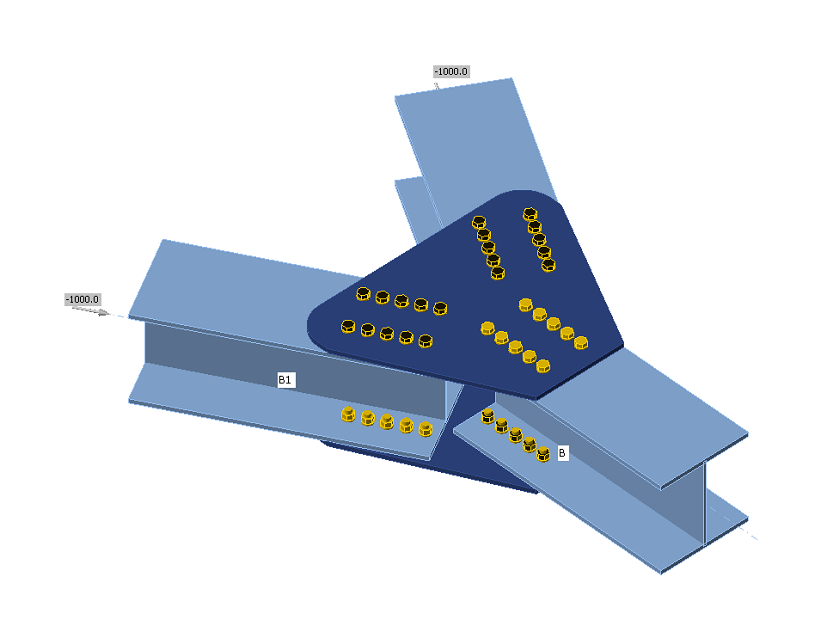### Choose language# Code-check of bolts and preloaded bolts (AISC)

$$The forces in bolts are determined by finite element analysis. The tensile forces include prying forces. The bolt resistances are checked according to AISC 360 - Chapter J3.BoltsTensile and shear strength of boltsThe design tensile or shear strength, ϕRn, and the allowable tensile or shear strength, Rn/Ω of a snug-tightened bolt is determined according to the limit states of tension rupture and shear rupture as follows:Rn = FnAbϕ = 0.75 (LRFD, editable in Code setup)Ω = 2.00 (ASD, editable in Code setup)where:Ab – nominal unthreaded body area of bolt or threaded partFn – nominal tensile stress, Fnt, or shear stress, Fnv, from Table J3.2The required tensile strength includes any tension resulting from prying action produced by the deformation of the connected parts.Combined Tension and shear in bearing type connectionThe available tensile strength of a bolt subjected to combined tension and shear is determined according to the limit states of tension and shear rupture as follows:Rn = F'nt Ab (AISC 360-16 J3-2)ϕ = 0.75 (LRFD, editable in Code setup)Ω = 2.00 (ASD, editable in Code setup)$$F'_{nt}=1.3 F_{nt} - \frac{f_{rv} F_{nt}}{\phi F_{nv}}$$ (AISC 360-16 J3-3a LRFD)$$F'_{nt}=1.3 F_{nt} - \frac{f_{rv} \Omega F_{nt}}{F_{nv}}$$ (AISC 360-16 J3-3b ASD)where: F'nt – nominal tensile stress modified to include the effects of shear stress Fnt – nominal tensile stress from AISC 360-16 Table J3.2 Fnv – nominal shear stress from AISC 360-16 Table J3.2 frv – required shear stress using LRFD or ASD load combinations. The available shear stress of the fastener shall be equal or exceed the required shear stress, frv Bearing strength in bolt holesThe available bearing strengths, ϕRn and Rn/Ω, at bolt holes are determined for the limit state of bearing as follows:ϕ = 0.75 (LRFD, editable in Code setup)Ω = 2.00 (ASD, editable in Code setup)The nominal bearing strength of the connected material, Rn, is determined as follows:For a bolt in a connection with standard holes:Rn = 1.2 lc t Fu ≤ 2.4 d t Fu (AISC 360-16 J3-6a, J3-6a, c)For a bolt in a connection with slotted holes:Rn = 1.0 lc t Fu ≤ 2.0 d t Fu (AISC 360-16 J3-6a, J3-6e, f)where: Fu – specified minimum tensile strength of the connected material d – nominal bolt diameter lc – clear distance, in the direction of the force, between the edge of the hole and the edge of the adjacent hole or edge of the material t – thickness of the connected material Preloaded boltsThe design slip resistance of preloaded class A325 or A490 bolt with the effect of tensile force FtPreloading force to be used AISC 360-10 tab. J3.1.Tb = 0.7 fub AsDesign slip resistance per bolt AISC 360-10 par. J3.8Rn = kSC μ Du hf Tb nsUtilization in shear [%]:Uts = V / ϕRn (LRFD)Uts = Ω V / Rn (ASD)where: As – tensile stress area of the bolt fub – ultimate tensile strength $$k_{SC}=1-\frac{F_t}{D_u T_b n_b}$$ – factor for combined tension and shear (LRFD) (J3-5a) $$k_{SC}=1-\frac{1.5 F_t}{D_u T_b n_b}$$ – factor for combined tension and shear (ASD) (J3-5b) μ – mean slip factor coefficient editable in Code setup Du = 1.13 – multiplier that reflects the ratio of the mean installed bolt pretension to the specified minimum bolt pretension hf = 1.0 – factor for fillers ns – number of the friction surfaces; Check is calculated for each friction surface separately V – shear force acting on the bolt ϕ = 1.0 – resistance factor for standard size holes (LRFD) editable in Code setup ϕ = 0.7 – resistance factor for slotted holes (LRFD) Ω = 1.5 – resistance factor for standard size holes (ASD) editable in Code setup Ω = 2.14 – resistance factor for slotted holes (ASD)$$